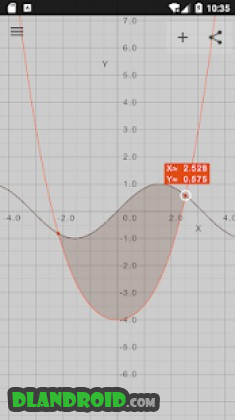Visual Math 4D - Graphical Calculator 5.3.1 Apk Full Paid latest | Download Android

# Visual Math 4D – Graphical Calculator 5.3.1 Apk Full Paid latest

event_note26 Feb 2019
android Apps, Education

Visual Math 4D – Graphical Calculator 5.3.1 Apk Full Paid latest is a Education Android app

Download last version Visual Math 4D – Graphical Calculator Apk Full Paid For Android with direct link

Visual Math 4D – Graphical Calculator is a Education android app made by appnova that you can install on your android devices an enjoy !

Visual Math 4D is a graphical calculator that allows you to visualize and solve your mathematical equations. It supports various types of equations, such as spherical, parametrical, polar and cartesian and implicit equations, which can be visualized and animated in 2D and 3D. You can also plot and animate vector fields in 2D and 3D.

Features:
– solve equations and visualize their intersections
– plot cartesian functions with intersections
– plot polar- and spherical functions
– plot parametrical equations
– plot complex functions (draw real part & imaginary part)
– plot vector fields in 2D and 3D
– plot implicit equations in 2D and 3D
– plot contours of your functions
– complex numbers
– vectors & matrices
– truth- and value tables
– trigonometrical and hyperbolic functions
– piecewise-defined functions
– logarithmic functions
– logical & binary operators
– definite integral
– n-th derivations
– statistical functions
– physical and mathematical constants with units
– animate variables
– share content with other apps
– no network connection required
– and a lot more…

The app is easy to use and helps students and engineers to visualize and solve their mathematical equations.Visual Math 4D – Graphical Calculator Apk Full

Whats New:

Updated contour plot and some bugfixes